# FV Function Excel

Calculates the future value of an investment

## What Is the Excel FV Function?

The FV Function Excel formula is categorized under Financial functions. This function helps calculate the future value of an investment.

As a financial analyst, the FV function helps calculate the future value of investments made by a business, assuming periodic, constant payments with a constant interest rate. It is useful in evaluating low-risk investments such as certificates of deposit or fixed rate annuities with low interest rates. It can also be used in relation to interest paid on loans.

### The Formula

=FV(rate,nper,pmt,[pv],[type])

This function uses the following arguments:

1. Rate (required argument) – This is the interest rate for each period.
2. Nper (required argument) – The total number of payment periods.
3. Pmt (optional argument) – This specifies the payment per period. If we omit this argument, we need to provide the PV argument.
4. PV (optional argument) – This specifies the present value (PV) of the investment/loan. The PV argument, if omitted, defaults to zero. If we omit the argument, we need to provide the Pmt argument.
5. Type (optional argument) – This defines whether payments are made at start or end of the year. The argument can either be 0 (payment is made at the end of the period) or 1 (the payment is made at the start of the period).

Make sure that the units of rate and nper are consistent. If we make monthly payments on a five-year loan at an annual interest of 10%, we need to use 10%/12 for rate and 5*12 for nper. If we make annual payments on the same loan, then we would use 10% for rate and 5 for nper.

### How to use the FV Function in Excel?

To understand the uses of the FV function in Excel, let’s consider a few examples:

#### Example 1 – FV function Excel

Let’s assume we need to calculate the FV based on the data given below: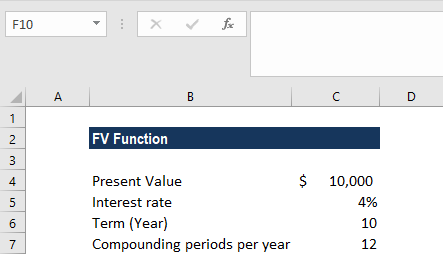The formula to use is: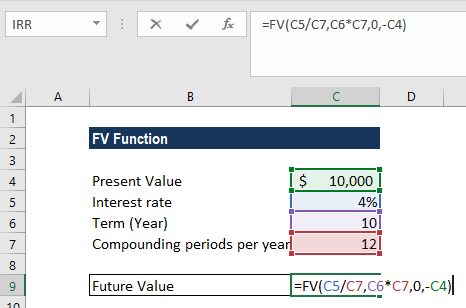As the compounding periods are monthly (=12), we divided the interest rate by 12. Also, for the total number of payment periods, we divided by compounding periods per year. As the monthly payments are paid out, they are entered into the function as negative values. If we forget to put the percent sign in cell C5, the calculation will be wrong. We need to enter the interest rate in percentage form or in decimal form, such as = 0.04.

We will get the result below: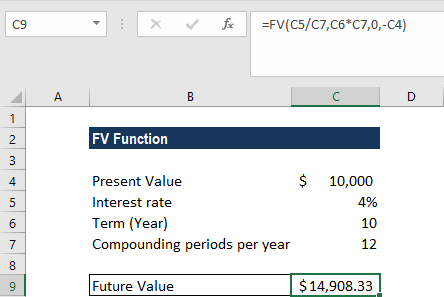#### Example 2 – FV function Excel

The example below shows how to use the function for personal finances. Assume we need to buy a car and are saving for it. The target is to buy in 1 year. There is \$10,000 in our bank account as savings. The bank pays 5% interest on our savings account. Our goal is to add \$2,000 for the next 12 months into the account. Let’s calculate the amount we would have at the end of the year to purchase the car.

Suppose we are given the information below: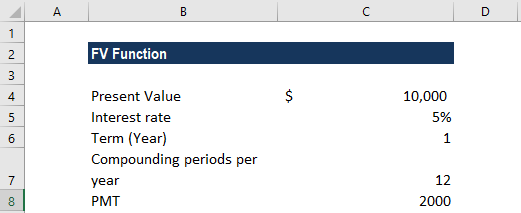The monthly contribution we can do is \$2,000. As this represents outflow, we took it as -2,000 (-C8).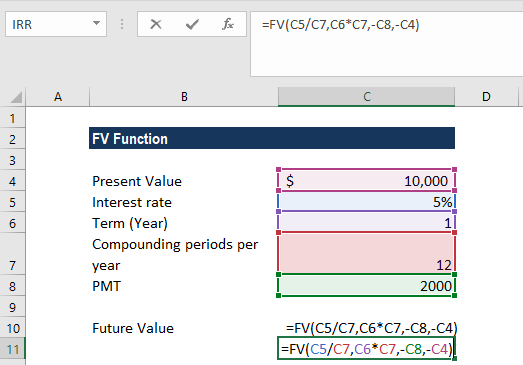We will get the following result: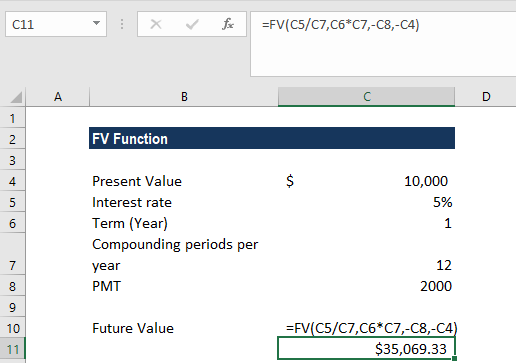### Things to remember about the FV Function

1. If the pmt argument is for cash going out of a business, the payment value will be negative. For cash received, it must be positive.
2. #VALUE! error – Occurs when any of the given arguments is non-numeric.

Thanks for reading CFI’s guide to important Excel functions! By taking the time to learn and master these functions, you’ll significantly speed up your financial analysis. To learn more, check out these additional CFI resources:

• Excel Functions for Finance